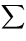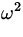# Rotational Kinetic Energy

The kinetic energy of rotation of a rigid body is obtained by first dividing it up into a collection of smaller masses, and then summing up the kinetic energies due to the tangential velocities of the individual masses making up that rigid body:
 KEr =(mivi2) =(miri2) =(miri2) =I. (12)
Note: The units of rotational kinetic energy are Joules (J).

When considering the total mechanical energy of a rigid body, this kinetic energy must be added to the kinetic energy of translation:

 KEt =mtotalvcm2 (13)

where vcm is the velocity of the center of mass.